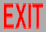# Developments inTHEORETICAL HIGH ENERGY PHYSICS

(an orientation talk on research for incoming graduate students, '02)

## Popular ≠ interesting

### Fundamental physics

#### → New concepts that can't be expressed in layman's terms

E.g.,
Special relativity → Minkowski space
Quantum mechanics → Hilbert space

I have been asked to describe research in high energy theory in popular terms. Such "couch potato physics" lacks all the flavor of what real physics is about, and reinforces all the popular misconceptions of science. (See, e.g., "Are you a quack?") Examples with which physics students are familiar are explanations of special relativity or quantum mechanics as found in television or popular nonfiction. The really fun things about fundamental physics are those that are so unfamiliar that they have no expression in everyday terms.

## More...

### Symmetry:

 Global → Local Internal U(1) (charge) → electromagnetism → SU(2) (isospin) → Yang-Mills → SU(N), etc. → Standard Model, GUTs Spacetime SU(2) (rotations) → general relativity → conformal → conformal gravity → supersymmetry → supergravity

### Dimensions:

 Internal ("d") particle (d=1) → string (d=2) → membrane (d=3) ↑ ↓ ↑ ↓ ↑ ↓ Spacetime ("D") D = 4 → D = 10 → D = 11 → twistor space (bosonic spinor coordinate) → superspace (bosonic + fermionic coordinates)

Many of the developments of the past half century are extensions of known concepts: in particular, enlarging symmetry groups, especially from global ones to local (independent transformations at each point in spacetime), thus replacing charge conservation with current conservation, which determines dynamics. Local symmetries are associated with forces, carried by particles of spin 1 and higher. Both internal (d) and spacetime (D) dimensions have also been enlarged: The particle, with a 1-dimensional worldline, extends to a string with a 2-dimensional worldsheet, which also requires 10 dimensions to propagate in, etc. Related generalizations are conformal symmetry, which uses twistor space, and supersymmetry, which uses superspace.

## Duality

(1) Change variables (masses, couplings, coordinates, momenta, fields)
(2) Expand in any (dimensionless) variable (depending on area of interest)

Lowest order (that variable → 0) = simpler theory: "classical"
Same quantum theory has different classical limits:

mechanics vs. field theory (wave-particle duality)
d = ?
D = ?
self-dual?

A topic that recently has received a great deal of attention is "duality", which is basically the identification of different classical formulations of the same quantum theory: Different actions, in terms of different variables, can lead to the same quantum theory. The usefulness of different formulations is that they lead to different JWKB expansions (perturbations in different "ħ's"). In particular, in string theory the D=10 superstring (d=2) was found dual to the D=11 supermembrane (d=3).